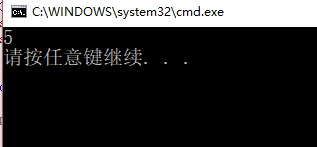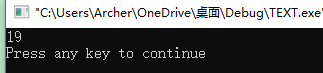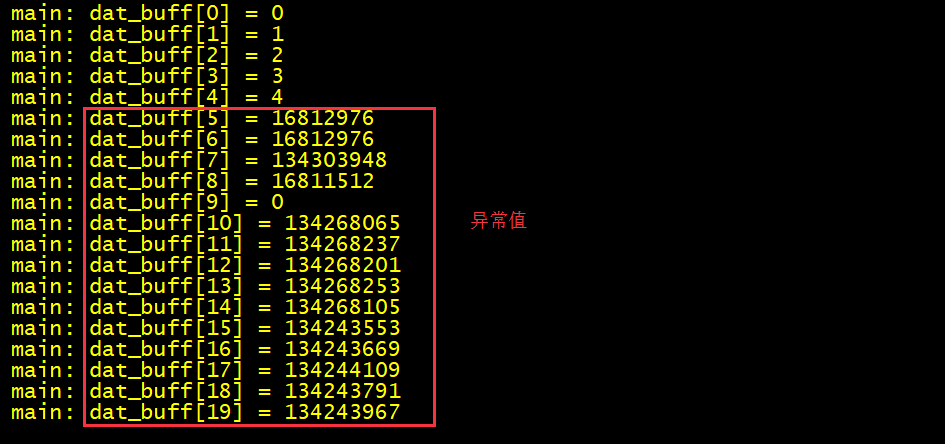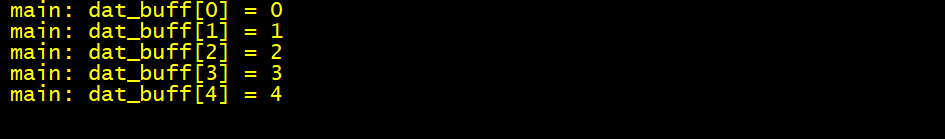• 求字符串长度 有两种方法 第一种： 非递归：思想很简单就是调用<string.h>库函数中的strlen()函数即可 代码如下所示： #include<stdio.h> #include<string.h> int main() { char *str = "hello...
求字符串的长度
有两种方法
第一种：
非递归：思想很简单就是调用<string.h>库函数中的strlen()函数即可
代码如下所示：
#include<stdio.h>
#include<string.h>
int main()
{
char *str = "hello";
int len = strlen(str);
printf("%d\n", len);
return 0;
}

第二种：
递归：思想就是大问题化成小问题，求字符串长度莫非就是字符串中的字母个数相加，例如hello就是5个字母即它的字符串长度就是5。递归满足的两个条件是：1.自己调用自己；2.终止条件
而怎么算把字符串读完，当遇到’\0’时就停止了，所以这个即是递归函数的终止条件；而怎么调用自己呢，我们知道字符串长度可以是h+(ello)的长度，而ello可以是e+(llo)的长度，以此类推，即可以写出递归函数
代码如下所示：
 #include<stdio.h>
#include<string.h>
Mystrlen(char *p)//p是指针变量存放的是字符串首位字符的地址
{
if (*p == '\0')//*p表示间接访问，若字符串地址里的内容是'\0'即表示字符串长度为0
{
return 0;
}
else//若字符串地址里的内容不是'\0',则最少返回1，即字符串中有一个字符
{
return 1 + Mystrlen(p + 1);
}
}
int main()
{
char *str = "hello";
int len = Mystrlen(str);
printf("%d\n", len);
return 0;

运行结果如下所示：展开全文• long length(char c[]); #include <stdio.h> int main(int argc, const char * argv[]) { long len,i; char str; for (i=0; i<30; i++) { ... printf("string has %ld characters.",l
long  length(char c[]);
#include <stdio.h>
int main(int argc, const char * argv[]) {
long len,i;
char str;
for (i=0; i<30; i++) {
scanf("%c",&str[i]);
}
len=length(str);
printf("string has %ld characters.",len);
return 0;
}
long  length(char c[])
{
char  *p=c;
while (*p!='#') {
p++;
}
return p-c;
}

output:
123vgvcuvcuiwbcvvugvujkbb jb#
string has 28 characters.Program ended with exit code: 0



展开全文• C语言求字符串长度 问题描述:求This is a C program字符串的长度: 问题分析:首先，了解字符串在C语言的定义，C语言中字符串是通过字符指针来间接实现的。利用一个循环来遍历该字符串，并且判断当前位置是否为空，...
C语言求字符串的长度
问题描述:求This is a C program字符串的长度:
问题分析:首先，了解字符串在C语言的定义，C语言中字符串是通过字符指针来间接实现的。利用一个循环来遍历该字符串，并且判断当前位置是否为空，在字符串的结尾，通常用\0来表示结束。
#include<stdio.h>
#include<string.h>
main()
{
int i=0;
char str="This Is a C Program";
while(str[i]!='\0')
{i++;}
printf("%d\n",i);
}


结果显示:展开全文算法
• 三、C语言求字符串长度 1. sizeof()与strlen()的区别 (1) sizeof是运算符，求变量、数组或者数据类型在内存中的实际长度，这是在编译阶段就已经确定了的一个数值。它的参数可以是变量[普通变量，指针变量]、数组...
上一篇：https://blog.csdn.net/qq_40088639/article/details/111562591

三、C语言求字符串的长度

1. sizeof()与strlen()的区别

(1) sizeof是运算符，求变量、数组或者数据类型在内存中的实际长度，这是在编译阶段就已经确定了的一个数值。它的参数可以是变量[普通变量，指针变量]、数组名或者数据类型。

示例程序1：

const char *str_ptr0 = "123456";

char ch_1 = '1';

char str_buff_2[]="123456789";

char str_buff_3="123456789";

int dat_buff_4={1,2,3,4,5};

int main(void)

{

printk("sizeof(str_ptr0) = %d", sizeof(str_ptr0));

printk ("sizeof(ch_1) = %d", sizeof(ch_1));

printk ("sizeof(str_buff_2) = %d", sizeof(str_buff_2));

printk ("sizeof(str_buff_3) = %d", sizeof(str_buff_3));

printk ("sizeof(dat_buff_4) = %d", sizeof(dat_buff_4));

return 0;

}

结果：

main: sizeof(str_ptr0) = 4           /* 指针变量，占4字节 */

main: sizeof(ch_1) = 1               /* char变量，1字节 */

main: sizeof(str_buff_2) = 10     /* 系统自动加上结束符，共占内存10字节 */

main: sizeof(str_buff_3) = 11     /* 定义时，就指定长度，占内存11字节 */

main: sizeof(dat_buff_4) = 20    /* 5个数，每个数用4字节来表示，共占20字节*/

示例程序2：

int main(void)

{

printk ("sizeof(int) = %d", sizeof(int));

printk ("sizeof(char) = %d", sizeof(char));

printk ("sizeof(int *) = %d", sizeof(int *));

printk ("sizeof(char *) = %d", sizeof(char *));

return 0;

}

结果：

main: sizeof(int) = 4

main: sizeof(char) = 1

main: sizeof(int *) = 4

main: sizeof(char *) = 4

sizeof()的参数可以是数据类型，所以还可以求构造类型[结构体、共用体和联合体]的长度及其变量的长度。

(2) strlen是一个函数，专门用于求字符串的长度，函数原型如下：

/**

*

* @brief Get string length

*

* @return number of bytes in string <s>

*/

size_t strlen(const char *s)

{

size_t n = 0;

while (*s != '\0') {

s++;

n++;

}

return n;

}

注意，它的参数是一个字符型指针。给定一个入口地址，它就会连续不停地遍历内存上的值，停止条件为：碰到结束符’\0’。所以，可以将字符数组的数组名作为参数，或者一个字符指针作为参数[这两者都代表字符串的首地址]。

示例程序：

const char *str_ptr0 = "123456";   //内存："123456\0"

char str_buff_1[]="123456";          //内存："123456\0"

char str_buff_2="123456";      //内存："123456\0\0\0\0\0"

int main(void)

{

printk("strlen(str_ptr0) = %d", strlen(str_ptr0));

printk ("strlen(str_buff_1) = %d", strlen(str_buff_1));

printk ("strlen(str_buff_2) = %d", strlen(str_buff_2));

return 0;

}

结果：

main: strlen(str_ptr0) = 6

main: strlen(str_buff_1) = 6

main: strlen(str_buff_2) = 6

2. 错误地使用sizeof求数组长度

2.1 对于字符型数组

如果使用sizeof求数组长度，则会包含最后一个结束符。

示例程序：

char str_buff_1[] = "123456"; //内存: "123456\0"

int main(void)

{

for (int i = 0; i < sizeof(str_buff_1); i++) {

printk("str_buff_1[%d] = %c",  i,  str_buff_1[i]);

}

return 0;

}

结果：

main: str_buff_1 = 1

main: str_buff_1 = 2

main: str_buff_1 = 3

main: str_buff_1 = 4

main: str_buff_1 = 5

main: str_buff_1 = 6

main: str_buff_1=       // sizeof(str_buff_1)长度为7，包含最后一个结束符

所以，对于字符数组，应该使用strlen来求得字符串的实际长度，程序修改如下：

示例程序：

char str_buff_1[] = "123456"; //内存: "123456\0"

int main(void)

{

for (int i = 0; i < strlen(str_buff_1); i++) {                // strlen(str_buff_1): 6

printk("str_buff_1[%d] = %c",  i,  str_buff_1[i]);

}

return 0;

}

结果：

main: str_buff_1 = 1

main: str_buff_1 = 2

main: str_buff_1 = 3

main: str_buff_1 = 4

main: str_buff_1 = 5

main: str_buff_1 = 6

2.2 对于非字符型数组

对于非字符型数组，使用sizeof求数组元素个数的时候，跟具体的类型有关，错误的使用示例程序：

int dat_buff={0, 1, 2, 3, 4};

int main(void)

{

for (int i = 0; i < sizeof(dat_buff); i++) {

printk("dat_buff[%d] = %d", i, dat_buff[i]);

}

return 0;

}

结果：因为sizeof(dat_buff)的结果是20 [ 5 * 4字节 ]，不是5，循环了20次。

正确求数组长度的方法：len = sizeof(数组名)/sizeof(元素类型)。

这个方法很好理解，sizeof(数组名)是编译阶段确定的内存大小，它是一片连续的内存块，大小等于数据个数(len)乘以每一个数据元素的字节大小。即：sizeof(数组名) = len * sizeof(元素类型)，所以：len = sizeof(数组名)/sizeof(元素类型)

程序修改后，如下：

int dat_buff={0, 1, 2, 3, 4};

int main(void)

{

for (int i = 0; i < sizeof(dat_buff)/sizeof(int); i++) {

printk("dat_buff[%d] = %d", i, dat_buff[i]);

}

return 0;

}

正确的运行结果：展开全文string
• C语言 strlen 函数用来求字符串的长度（包含多少个字符）。 strlen() 函数从字符串的开头位置依次向后计数，直到遇见’\0’，然后返回计时器的值。最终统计的字符串长度不包括’\0’。 头文件：string.h 二、使用...
• ## C语言获取字符串长度的几种方法

千次阅读 多人点赞 2020-09-02 10:50:58
C语言中，想要获取字符串长度可以有很多方法，下面分别介绍 一、使用sizeof()运算符 在C语言中，sizeof() 是长度...在使用sizeof()求字符串长度时，会将 ‘\0’ 也计算到字符串长度中。例如"abcd"用sizeof()求长度会
• C语言 计算字符串长度的几种方法 C语言计算字符串长度，可以手动计算也可以使用库函数或者sizeof()操作符。 自定义函数长度 使用strlen()函数 使用sizeof()操作符 自定义函数 int cont_str(char *s) { int i = 0;...
• #include&lt;stdio.h&gt;int lenstr(const char* str){ int i=-1; while(str[++i]); return i;}int main(){ char p; printf("...//输入字符串 gets(p); printf("string len:%d...c++
• C语言计算字符串长度的几种方法 C语言计算字符串长度，可以手动计算也可以使用库函数或者sizeof()操作符。 自定义函数长度使用strlen()函数使用sizeof()操作符 自定义函数 int cont_str(char *s) { ...
• #include<stdio.h> int main(){ int i; char arr; int len; while(scanf("%s",arr)!=EOF){ len=0; for(i=0;arr[i]!='\0';i++){ len++; } printf("%s:%d",arr,len); } ...return ...
• 常见的计算长度的函数有两个，sizeof（）和strlen（），但是，它们之间的差别还是很大的。 sizeof（）：主要负责计算数组的长度； strlen（）：主要负责...（2）strlen（）：strlen，求字符串有效长度 例如： #in...
• 说到字符串这个老铁啊，它有一个明显的特征，就是以‘\0’结尾。那么如果要求它的长度，就可以利用这个特性。 下面贴出它的代码实现：（此处代码可正确运行，但是并不是最终结果哦，最终结果后面给出） #...
• 2417: C语言习题 字符串长度 时间限制:1 Sec内存限制:128 MB 提交:758解决:548 题目描述 写一函数，一个字符串的长度。在main函数中输入字符串，并输出其长度。 输入 一行字符串 ...
• 主要介绍了C语言求字符串长度的函数的几种实现方法,需要的朋友可以参考下
• 一个长度不大于100的字符串的长度，要求不使用strlen方法，通过自定义的函数实现字符串长度的计算。  函数定义为：int GetStrLen(char *pStr) Input 一行字符串，使用gets(str)方法读取此行字符串。 Output ...
• Problem A: C语言习题 字符串长度 Time Limit: 1 Sec Memory Limit: 128 MB Submit: 1612 Solved: 1192 [Submit][Status][Web Board] Description 写一函数，一个字符串的长度。在main函数中...
• 在C/C++语言中没有专门的字符串变量，通常用字符数组来存放字符串。字符串是以“\0”作为结束...● 测字符串长度函数strlen。字符串是面试的重点考查部分的相关知识，通过考查字符串的相关知识可以考察程序员的编程...
• //在不创建临时变量的情况下字符串长度 //关键点在于指针指向数组的首元素，指针向前进的同时带着一个计数器，在指针前进的同时计数，指针在遇到/0时停下来 #include<stdio.h> #include<string.h>......

# c语言求字符串长度c语言 订阅